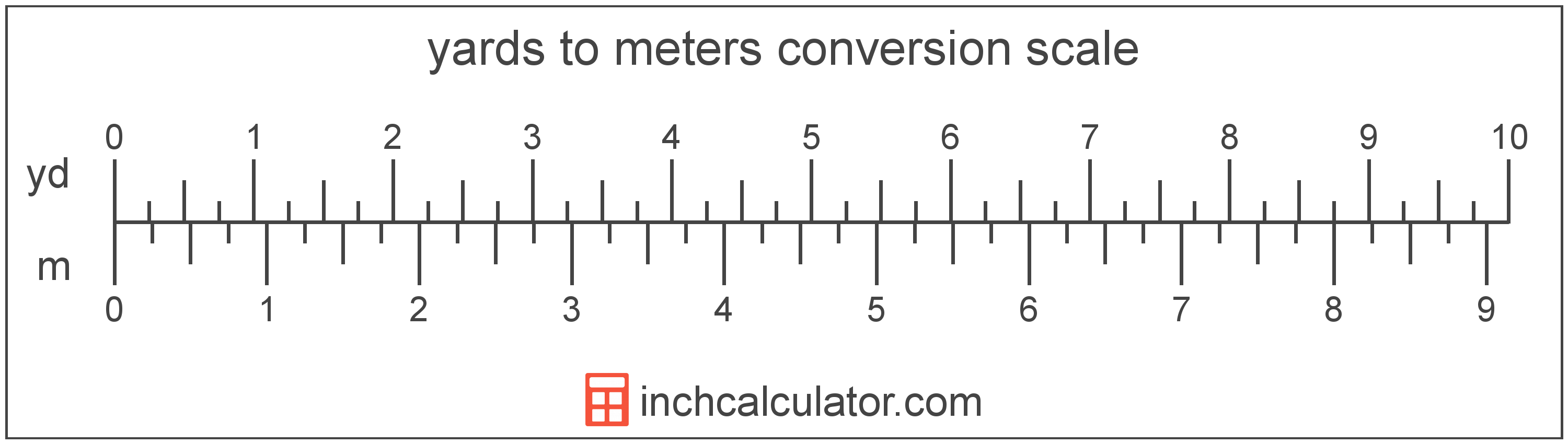# Meters to Yards Converter

Enter the length in meters below to get the value converted to yards.

Results in Yards:1 m = 1.093613 yd
1 m = 1 yd 0.28 ft

Do you want to convert yards to meters?

## How to Convert Meters to Yards

To convert a measurement in meters to a measurement in yards, multiply the length by the following conversion ratio: 1.093613 yards/meter.

Since one meter is equal to 1.093613 yards, you can use this simple formula to convert:

yards = meters × 1.093613

The length in yards is equal to the length in meters multiplied by 1.093613.

For example, here's how to convert 5 meters to yards using the formula above.
yards = (5 m × 1.093613) = 5.468066 yd### How Many Yards Are in a Meter?

There are 1.093613 yards in a meter, which is why we use this value in the formula above.

1 m = 1.093613 yd

## What Is a Meter?

According to the most recent 2019 definition, the meter is defined as the distance traveled by light in vacuum during a time interval with a duration of 1/299,792,458 of a second. One meter is equal to 100 centimeters, 3.28084 feet, or 39.37 inches.

The meter, or metre, is the SI base unit for length in the metric system. Meters can be abbreviated as m; for example, 1 meter can be written as 1 m.

## What Is a Yard?

The yard is a unit of length measurement equal to 3 feet or 36 inches. The international yard is legally defined to be equal to exactly 0.9144 meters.

The yard is a US customary and imperial unit of length. Yards can be abbreviated as yd; for example, 1 yard can be written as 1 yd.

We recommend using a ruler or tape measure for measuring length, which can be found at a local retailer or home center. Rulers are available in imperial, metric, or a combination of both values, so make sure you get the correct type for your needs.

Need a ruler? Try our free downloadable and printable rulers, which include both imperial and metric measurements.

## Meter to Yard Conversion Table

Table showing various meter measurements converted to yards.
Meters Yards
1 m 1.0936 yd
2 m 2.1872 yd
3 m 3.2808 yd
4 m 4.3745 yd
5 m 5.4681 yd
6 m 6.5617 yd
7 m 7.6553 yd
8 m 8.7489 yd
9 m 9.8425 yd
10 m 10.94 yd
11 m 12.03 yd
12 m 13.12 yd
13 m 14.22 yd
14 m 15.31 yd
15 m 16.4 yd
16 m 17.5 yd
17 m 18.59 yd
18 m 19.69 yd
19 m 20.78 yd
20 m 21.87 yd
21 m 22.97 yd
22 m 24.06 yd
23 m 25.15 yd
24 m 26.25 yd
25 m 27.34 yd
26 m 28.43 yd
27 m 29.53 yd
28 m 30.62 yd
29 m 31.71 yd
30 m 32.81 yd
31 m 33.9 yd
32 m 35 yd
33 m 36.09 yd
34 m 37.18 yd
35 m 38.28 yd
36 m 39.37 yd
37 m 40.46 yd
38 m 41.56 yd
39 m 42.65 yd
40 m 43.74 yd

## References

1. International Bureau of Weights and Measures, The International System of Units, 9th Edition, 2019, https://www.bipm.org/documents/20126/41483022/SI-Brochure-9-EN.pdf
2. National Bureau of Standards, Refinement of Values for the Yard and the Pound, https://www.ngs.noaa.gov/PUBS_LIB/FedRegister/FRdoc59-5442.pdf